# Trigonometric Planes Menu Help

#### Fractal Zoomer

Trigonometric Planes Menu
•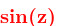, transforms the initial complex number, by using the transformation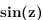.
•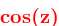, transforms the initial complex number, by using the transformation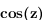.
•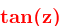, transforms the initial complex number, by using the transformation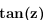.
•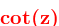, transforms the initial complex number, by using the transformation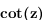.
•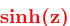, transforms the initial complex number, by using the transformation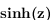.
•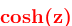, transforms the initial complex number, by using the transformation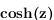.
•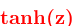, transforms the initial complex number, by using the transformation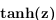.
•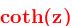, transforms the initial complex number, by using the transformation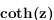.
•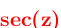, transforms the initial complex number, by using the transformation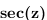.
•, transforms the initial complex number, by using the transformation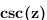.
•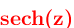, transforms the initial complex number, by using the transformation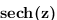.
•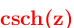, transforms the initial complex number, by using the transformation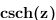.